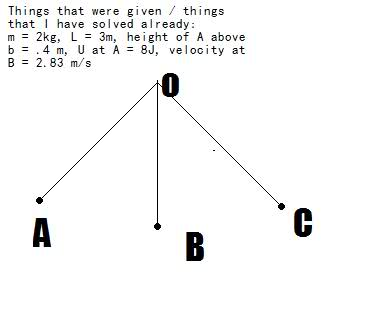# Ball on a pendulum question

• ah19

## Homework Statement

A 2 kg ball on a 3 meter long string swings along patch ABC. Angle AOB and AOC equal 30* each. Find the acceleration of the ball at point A.## Homework Equations

Guesses: F=ma, v = vo + at

## The Attempt at a Solution

I tried using tan30 = X/2.6 and solving for X to find the horizontal distance. Then, draw a FBD and mg = 20 and the other leg is X, which would allow me to solve for the hypotenuse. But I realized that I cannot add a force with a distance.

Hint:
tangential acceleration in point A,since there is no radial acceleration cause V=0(ar=V^2/R)
Goodluck.
Edit:
hint number 2
find the angle of the component of the force mg in the tangential direction using trigo(height ,Length)

Hint:
hint number 2
find the angle of the component of the force mg in the tangential direction using trigo(height ,Length)

hi,

how do i do that, i tried to see if the triangles were similar but no luck